# Questioning the Magic of Simpson’s Rule

Arif Zaman

Lahore University of Management Sciences

Abstract: Given a function evaluated on a finite equally spaced grid of points, Simpson’s rule provides an estimate of the definite integral of the function. The form of the estimate is a weighted average of the function values, with the weights alternating between high and low. It is difficult to come up with a reasonable explanation of why the weights should alternate this way, but the extraordinary accuracy of the method silences doubters. Here we propose alternatives to Simpson’s rules which avoid the alternating weights, and provide some explanation of the alternation of Simpson’s rule.

Keywords: Quadrature, Simpson’s Rule, Numerical Integration.

AMS Subject Classification: 65D32

## The Absurdity of Simpson’s RuleIntroductory calculus texts, numerical analysis texts, mathematical handbooks and even some high school texts often start the topic of numerical integration by considering a function f that has been evaluated along an equally spaced grid of n+1 points (see Figure 1) starting from a and ending at b with spacing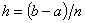. Let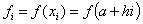be the function values along the grid. When n is an even number, the definite integral of f is approximated by the extended Simpson’s rule,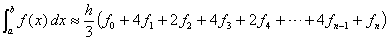.                  (1)

This result is a weighted sum of the function values, but the alternating sequence of weights in this equation often comes as a surprise not only to students but often to teachers of the subject as well. The weights suggested by Simpson’s rule seem intuitively unreasonable. Why should every even point (other than the endpoints) have half the weight of the odd points? What makes them different? Especially if we were to consider that to integrate from a+h to b+h the formerly odd points would now be even, and the even ones would be odd.

While this is not a statistical problem, the values of the function do have some similarities to observations from an unknown population, and the integral does correspond to a population average. Statistical wisdom suggests that the simple mean with equal weights is the “best” estimate for an unknown population mean, and amongst all other linear estimates, it has the minimum variance. In a statistical sample, giving half of the sample points a weight of 4/3 and the other half a weight of 2/3 (ignoring the endpoints) would have the same accuracy as a simple mean with a sample size that was 10% smaller.

## Explaining Simpson’s Rule

The extended Simpson’s rule can be seen as one of the class of extended Bode’s rules, or as an intermediate step in Romberg extrapolation.

### Simpson’s Rule as a Polynomial Fit

Given the set of n+1 function values, one approach to estimating the integral is to fit a polynomial of degree n and then integrating the fitted polynomial. The function values at the grid points give n+1 linear equations in the n+1 polynomial coefficients. The integral is also a linear function of the polynomial coefficients. So the definite integral can be written as a linear function of the function values. This is the Bode’s n+1-point rule,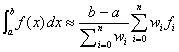.                                             (2)

with the sequence of weights wi given in Table 1.

 n Table 1: Weights wi for Bode’s n+1 point rule 1 1, 1 2 1, 4, 1 3 1, 3, 3, 1 4 7, 32, 12, 32, 7 5 19, 75, 50, 50, 75, 19 6 41, 216, 27, 272, 27, 216, 21 7 751, 3577, 1323, 2989, 2989, 1323, 3577, 751 8 989, 5888, –928, 10496, –4540, 10496, –928, 5888, 989

For n=1, this turns out to be the well known trapezoidal rule, while for n=2 this is the Simpson’s rule. Notice that the weights become more non-uniform as the degree of the polynomial fit increases, to the point that when n=8, we start getting negative weights!

Extended forms of Bode’s rules are defined when the number of intervals n can be factored into jm. For each of the j groups of m consecutive intervals, we can apply the m+1 point Bode’s rule and sum the result to get the overall integral. It is easily verified that the extended Simpson’s rule in equation 1, is obtained from the extension of Bode’s rule for n=2.

### Simpson’s Rule as an Accelerated Limit

If we represent the n point extended trapezoidal rule by T0(n),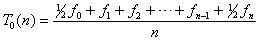,                                   (3)

then the sequence T0(2n) will approach the integral, by successive refinements of the same grid. Romberg integration tries to improve the convergence rate of this series by using Richardson extrapolation. The answer is best computed using the recursive formula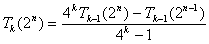,                                         (4)

with Tn(2n) being the final answer. The following table illustrates the recursion

 k=0 1 2 3 L n=1 T0(1) 2 T0(2) T1(2) 4 T0(4) T1(4) T2(4) 8 T0(8) T1(8) T2(8) T3(8) M M M M M O

The first column, for k=0, is obtained by successive trapezoidal rules. The next column, with k=1, is the first improvement and is easily be seen to be equivalent to the extended Simpson’s rule.

## Competing with Simpson’s Rule

If we want to come up with a “better” or “more reasonable” rule than Simpson’s, we need to define what is meant by better. Simpson’s rule is often used as a general purpose integration routine, which it is absolutely not suited for. The assumption of existence of many high order bounded derivatives is essential for the procedure to work. The accuracy of the extended Simpson’s rule is given by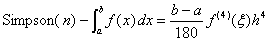(5)

for some ξ in (a, b). Note that Simpson’s rule will perform no better than a simple trapezoidal rule for cubic splines unless care is taken to align the knot points with the grid, because splines have a discontinuous third derivative, and unbounded fourth derivative. For such functions, general purpose adaptive integration schemes perform much better, but narrowing in on discontinuities and asymptotes.

For smooth analytic functions evaluated on a number of points, Romberg extrapolation, higher order Bode’s rules or Gaussian quadrature can do much better.

### A flat competitor

Let us consider another way to approximate the same integral. Break the integral into three pieces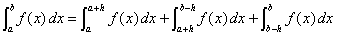.                         (6)

Use the extended Simpson’s rule for the middle integral to get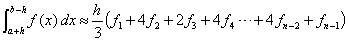.                        (7)

Note that the weights in equation ‎6 are now reversed as compared to the weights of equation ‎1. For the two end integrals, if we use three point quadratic approximations we get.                                         (8)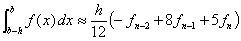.                                     (9)

Substituting equations ‎6‎, 7, ‎8 into equation ‎5 gives another formula for the same integral, with weights that are nearly opposite those of the extended Simpson’s rule, namely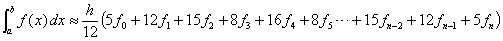.  (10)

The average of the extended Simpson’s rule (equation ‎1) and this modified one (equation ‎9) results in the approximation(11)

Because of its construction, we can guarantee that it integrates quadratics exactly, but unlike the extended Simpson’s rule, the weights of all the points are the same except for the final three points at each end. We will refer to equation ‎10 as the flat rule, and evaluate its performance relative to Simpson’s rule.

## The defeat of the flat rule

For our flat construction, equations ‎7 and ‎8 each has errors of size h4f(3)(ξ)/24, while the central portion is an application of the extended Simpson’s rule, and so by equation ‎11, it has an error of (ba–2h)h4f(4)(ξ)/180. The combined error is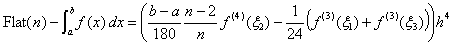(12)

ξ1 in (x0, x0+h), ξ2 in (x1, xn–1) and ξ3 in (xn–1, xn).

It is surprising to see that the two intervals of size h in equations ‎7 and ‎8 each have an error that is of the same order of magnitude as the entire error of the n–2 remaining intervals. Thus while the flat rule performs at the same order of magnitude error as Simpson’s rule, the constant is worse.

### A different analysis and derivation for the flat rule

Instead of breaking the (a, b) interval into n subintervals at the grid-points as before, we break it into the n+1 intervals at the midpoints of the grid (x0, x0+h/2), (x1h/2, x1+h/2), (x2h/2, x2+h/2)…(xnh/2, xn). For each interval, we can once again use a three point quadratic fit.                          (13)

for some ξ2 in the interval (xih/2, xi+h/2). This error is nearly half that of the Simpson’s rule. For the half intervals at the ends, we will need approximations of the form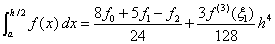,                               (14)

and(15)

for appropriate values of ξ1 and ξ3 in their respective intervals. Interestingly, adding equations ‎13‎, 14‎ and 15 together results in exactly the same weights as the flat rule first found in equation ‎10! On the other hand, because of the different derivation the total error is given by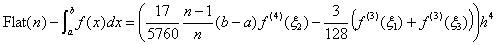(16)

for some ξ1 in (x0, x0+h/2), ξ2 in (x1h/2, xnh/2) and ξ3 in (xnh/2, xn). Equation ‎16 gives a tighter bound than equation ‎12, but the conclusion remains unchanged.

## The Magic of Simpson’s Rule

### Its done with mirrors

It is well known (and obvious from Equation ‎11) that even though the derivation of Simpson’s rule used a quadratic fit, Simpson’s rule integrates cubic curves exactly. An interesting consequence of this is that for n=2 when we have three function values, no advantage can be obtained by measuring the value of the function at any other location. This fact is best seen by a simpler analogy of the midpoint rule. On the (a, b) interval suppose only the value fm = f(xm) is known, for xm = (a+b)/2. The only reasonable estimate for the integral seems to be (ba) fn using the constant (0th degree polynomial) fit. If we were given the additional information that fn = f(xn), we can now use a linear (1st degree polynomial) fit, but the value of the integral will be the same (ba) fn regardless of the slope of the line. In an analogous manner, if a fourth point is given where n=2, no matter what cubic equation is fit through the four points, the integral will still be given by Simpson’s rule using only three points.

The symmetry of the points in both cases guarantees that odd functions will integrate exactly. This means that all Bode’s rules with an odd number of points n+1 get one extra degree of accuracy and are exact for polynomials of degree n+1.

Bode’s rules are easily derived as solutions of n+1 linear equations. If we relax the constraint of the grid of points being equally spaced, the position of the points gives another n+1 degrees of freedom. This is called Gaussian Quadrature, and allows exact integration of polynomials up to degree 2n+1, although the equations to solve become non-linear.

The three point Gaussian Quadrature places one point in the center of the interval and two other points symmetrically placed inside the interval.

## The special case of Simpson’s rule

There are two special properties of Simpson’s rule. One is that because of the symmetric placement of the three gridpoints, with the center point coinciding with the Gaussian point, one extra degree of accuracy is obtained in the polynomial. The second is that by using an equally spaced grid in the extended Simpson’s rule, the endpoints are shared, so that with n+1 points, we can perform n/2 Simpson’s rules, and not (n+1)/3.

What this means is that if we tried to outperform the extended Simpson’s rule using an extended 2 point Gaussian quadrature, the Gaussian quadrature would be exact up to  3rd degree polynomials (the same as Simpson’s rule), and would use two points per interval, once again similar to Simpson’s rule. Of course higher degree quadrature can be used to get better results.

## A fresh approach

With n+1 points, and the constraint that cubic equations must integrate exactly is only 4 constraints. That leaves a lot of degrees of freedom. To come up with a specific solution, the approach we have been using is to use some physical justification. One can simply constrain the solution to have what we consider to be desirable properties. One example is to minimize the sum of squared weights. A simpler one is to force all the middle values to be equal. When we do the latter, we get a rule that is very simple in form

w0 = wn = n/(2n + 2)

wi = n2/(n2 – 1) for all in.

This can be seen as a modification of the simple trapezoidal rule

[Trapez(n) – (f0 + fn)/2n] n2/(n2–1)

Unfortunately its error is of order h2 not h4.

[(ba)3/720] h2

We can try to modify the last two terms of the sequence which then yields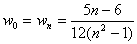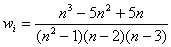for i ≠ 0, 1, n–1, n.

This can be rewritten as